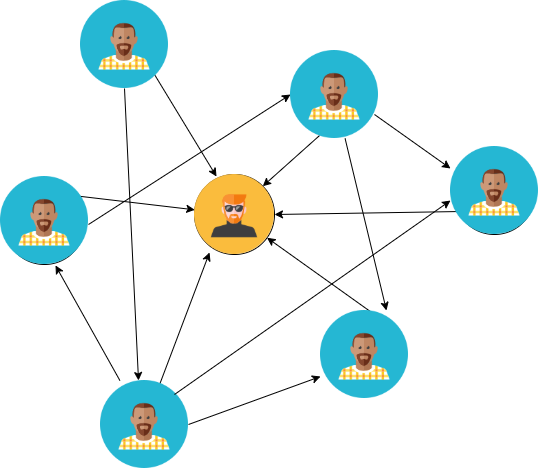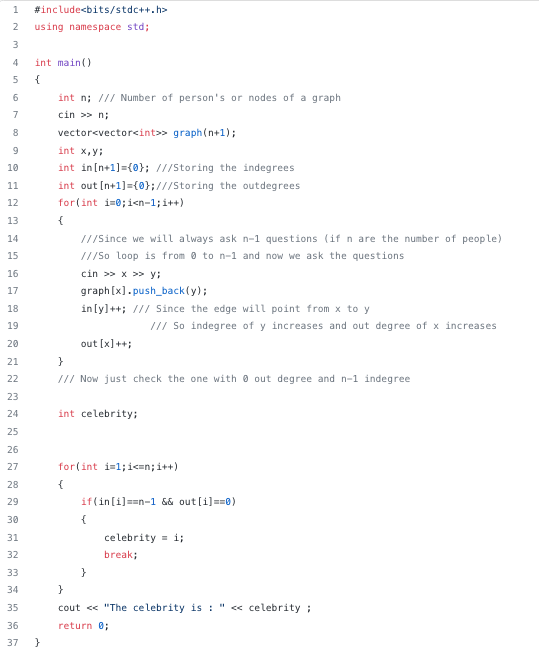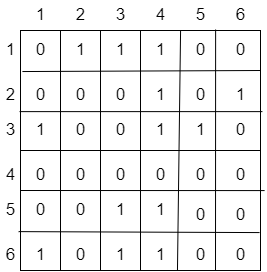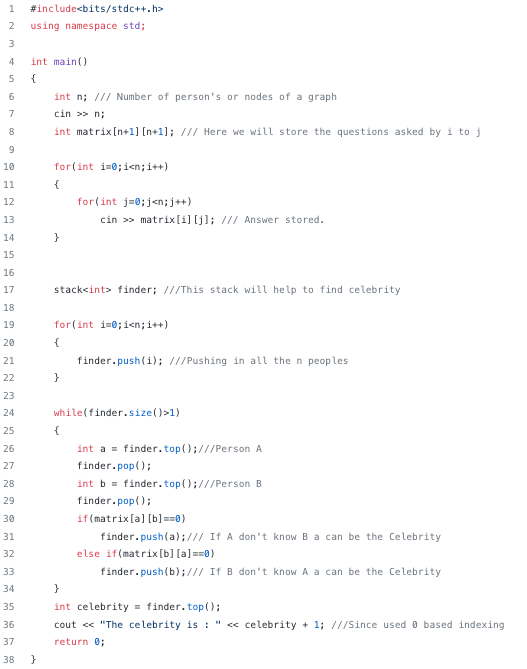# The Celebrity Problem

### Problem Statement

There are n+1 people at a party. They might or might not know each other names. There is one celebrity in the group, and the celebrity does not know anyone by their name. However, all the n people know that celebrity by name.

You are given the list of people present at the party, and we can ask only one question from each one of them. “Do you know this name”? How many maximum numbers of questions do you need to ask to identify the actual celebrity?

### Solution approach 1: Recursive approach using decrease and conquer

This problem can be solved by reducing the problem size by one — decrease and conquer strategy, a recursive technique helpful in solving many problems. In the decrease and conquer strategy, we reduce the problem size by one (or sometimes by more than one) and then recursively apply the same strategy to the reduced problem till the problem size becomes small. Let’s understand this by solving the celebrity problem.

Among n person, we start by choosing 2 persons, say A and B and ask A whether he/she knows B. If “Yes”, then A cannot be a celebrity and can be eliminated. If “No”, then B cannot be a celebrity and can be eliminated. This reduces the problem size by one. We repeat the same strategy among the group of n persons. After n questions, one person (say X) will remain. It is given that, there is a celebrity present at the party then it should be this person (why? think!). So we have to ask a maximum of n questions to find the celebrity.

Suppose it is given that a celebrity may not be present at the party, then what would be the maximum questions we ask to identify a celebrity or determine that a group has no such person? The idea would be simple: from the above approach, if there is a celebrity, then it should be the last remaining person after n number of questions(why? think!). To check whether person X is a celebrity, we ask X whether he knows everybody else and whether everybody else knows X. Total number of questions = n + n + n = 3n.

### Solution approach 2: Modeling and solving the celebrity problem using graph

Whenever there occurs some problem of connections or some relationship we can model that problem in terms of a graph? Can we convert this problem into a graph problem? Well, yes indeed it is a problem of graph which you can be visualized by the diagram below.The person in the middle is the celebrity

The image has been represented as an undirected graph where the in-degree represents the number of people who know him and the out-degree represents the number of people he knows. So by assigning all the indegrees and out-degree if we could find the one with zero outdegrees and n in-degree would be our celebrity. Now let's see how can we code it.

Solution code C++### Solution approach 3: Modeling and solving the celebrity problem using matrix and stack

Now in this approach, we will see the beauty of stack and matrix representation. We try to represent the situation in the boolean matrix and then we will use the power of stack to reach to celebrity. To analyze this problem in this approach we will require an n*n matrix if we have n people. Now let ‘i’ represent the row and ‘j’ column. Then any cell (i, j) will represent whether i know j or not. If a cell contains 1 means yes i know j otherwise No.

The matrix after filling in the informationAfter getting this information we will process it using stack. Firstly, we will store all n people in the stack and each time we will pick up two-person A and B and ask questions about whether A knows B or not, and this time we will simultaneously ask B whether he/she knows A or not. If both say ‘Yes’ We will not put them back to stack and if one of them say’s ‘No’ we will put him/her to stack. In the end, we will have only two-person remaining and thus we will get the celebrity there. Let’s see how can we code this out.

Solution code C++### Critical ideas to think!

• In the first approach, can we improve the above algorithm to 3n — 1 number of questions? Can we think of an even better algorithm? It can be shown that it can be done using 3n − log n questions and this is the best possible solution! In other words, one cannot solve this problem using a lesser number of questions.
• How can we design working code for the first approach?
• Can we think to solve this iteratively using two-pointers?
• What would be the time and space complexities of the above approaches?
• Can we think to solve this problem using some other data structures?

Hope you enjoyed this blog. Enjoy learning, Enjoy thinking!